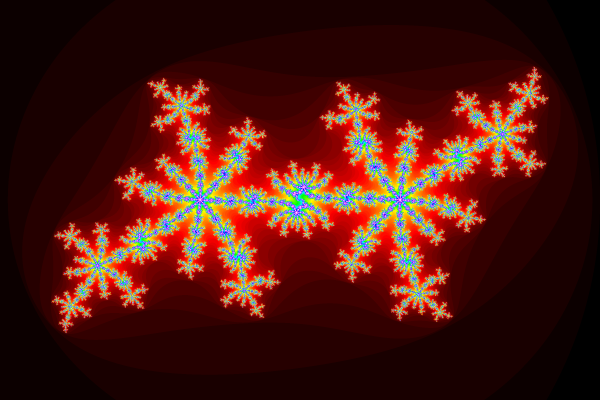# March 2013

## Friday, the 1st## Sunday, the 3rd

This is, possibly, the cleanest numpy version of a fractal generator. Weeell, not the cleanest because I put in a custom color map, since I like to be able to choose between several.

This is not what I used for my previous entry, but it's reasonably fast as long as you want just one file. I'll still have to do some tests to figure out what's best to use for movies. And I don't have the time to do that now.

import numpy as np
import matplotlib
import matplotlib.cm as cm
import matplotlib.pyplot as plt

def mandelbrot(
filename = 'mandelbrot',
max_iter = 50,
x0 = -2., x1 = 1.,
y0 = -1.5, y1 = 1.5,
dpi = 2**7,
colormap = cm.bone):
xvals = np.arange(x0, x1, 1./dpi, dtype = np.float32)
yvals = np.arange(y0, y1, 1./dpi, dtype = np.float32)
z0 = xvals[np.newaxis, :] + 1j*yvals[:, np.newaxis]
z = z0.copy()
iterations = np.zeros(z.shape, dtype = np.int)
for i in range(max_iter):
good_indices = np.abs(z) < 2
iterations[good_indices] += 1
z[good_indices] = z[good_indices]**2 + z0[good_indices]
fig = plt.figure(figsize = (x1-x0, y1-y0))
ax = fig.add_axes([.0, .0, 1., 1.])
ax.set_axis_off()
ax.imshow(iterations,
cmap = colormap,
interpolation = 'none',
rasterized = True)
fig.savefig(filename + '.png', format='png', dpi=dpi)
return None

def main():
cdict1 = { 'red'  : ((0.0, 0.0, 0.0),
(0.8, 0.0, 0.0),
(1.0, 1.0, 1.0)),
'green': ((0.0, 0.0, 0.0),
(0.4, 0.0, 0.0),
(0.8, 1.0, 1.0),
(1.0, 1.0, 1.0)),
'blue' : ((0.0, 0.0, 0.0),
(0.4, 1.0, 1.0),
(1.0, 1.0, 1.0)),
}
ice_map = matplotlib.colors.LinearSegmentedColormap('ice_map', cdict1)
mandelbrot(
filename = 'bla',
dpi = 2**8,
colormap = ice_map)
return None

if __name__ == '__main__':
main()


By the way, I used pygmentize from pygments for generating the html version of the code. Apparently it can generate css styles etc, but for now I told it to just put in all the colors explicitly, even though it dutifully informed me that the file would become kind of big. It was a bit more work than using verbments though...

## Saturday, the 9th

at some point during highschool I had dreams about quake and maybe half-life. it makes sense. I had time to play those games.

during college, I had a couple of dreams about spider. I'm not sure why they weren't nightmares, there was this great big green background and some cards moving around on it. but it was ok. I guess this speaks volumes about my passion for learning...

last night I dreamt about git and todo.

it's fun. as a kid, I had a lot of ideas about what it would be like to grow older. and, sometimes, it does feel like I'm getting closer to some pattern of average dude''. but I'm still dumbstruck sometimes as to how I am, in fact, getting weirder. and delighted.

anyway, I gotta get back to work. I just felt like sharing.

## Saturday, the 16th

$0 = e^{\imath \pi} + \sum_{n=1}^\infty \frac{1}{2^n}$

yes, I caved and I started using mathjax for displaying latex math. yes, it is javascript and people should not use javascript. but the alternative was to generate many many png files, which might become a very big headache. and, anyway, I can hope that in the future browsers will know how to handle latex by themselves.

I did this because I wanted an easy way to talk about centered differences. The group is working on something where we need to compute third order derivatives in the database (turbulence database, click the previous link for more info). So I told them I'd provide a general formula for centered differences...

well, the formula is formally simple: $F^{(k)}_n [i] \approx \frac{1}{h^k}\sum_{j = -n}^n c^n_{kj} F[i+j]$ where $$n$$ is the number of neighbours taken into account, $$k$$ is the order of the derivative, $$h$$ is the distance between grid points and $$i$$ is the grid point where you need the derivative. for 3D, you just need a tensor product: $F^{(k_x, k_y, k_z)}_n [i_x, i_y, i_z] \approx \\ \frac{1}{h_x^{k_x}h_y^{k_y}h_z^{k_z}} \sum_{j_x = -n}^n \sum_{j_y = -n}^n \sum_{j_z = -n}^n c^n_{k_xj_x} c^n_{k_yj_y} c^n_{k_zj_z} F[i_x+j_x, i_y + j_y, i_z + j_z]$ and the coefficients $$c^n_{kj}$$ are found very easily with any symbolic computation tool. I used sympy to get them with this small piece of code.